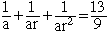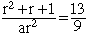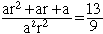Tashalee student The sum of the first 3 terms of a geometric series is 13. The sum of their reciprocal is 13/9. how do you find the first three terms? Hi Tashalee, Since you have a geometric series the first three terms can be written a, ar, ar2 for some numbers a and r. Thus you have a + ar + ar2 = 13 andorMultiplying the numerator and denominator br a givesSince a + ar + ar2 = 13 a2r2 = 9 and thus ar = 3 or a = 3/r. Substitute into the above equation and solve for r. Cheers, Penny Go to Math Central